# Difference between revisions of "Sine-squared function"

This article is about a particular function from a subset of the real numbers to the real numbers. Information about the function, including its domain, range, and key data relating to graphing, differentiation, and integration, is presented in the article.
View a complete list of particular functions on this wiki

## Definition

This function, denoted$\sin^2$, is defined as the composite of the square function and the sine function. Explicitly, it is the map:$x \mapsto (\sin x)^2$

For brevity, we write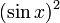$(\sin x)^2$ as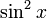$\sin^2x$.

## Key data

Item Value
Default domain all real numbers, i.e., all of$\R$
range$[0,1]$, i.e.,$\{ y \mid 0 \le y \le 1 \}$
absolute maximum value: 1, absolute minimum value: 0
period$\pi$, i.e.,$180\,^\circ$
local maximum value and points of attainment All local maximum values are equal to 1, and are attained at odd integer multiples of$\pi/2$.
local minimum value and points of attainment All local minimum values are equal to 0, and are attained at integer multiples of$\pi$.
points of inflection (both coordinates) odd multiples of$\pi/4$, with value 1/2 at each point.
derivative$x \mapsto \sin(2x) = 2\sin x \cos x$, i.e., double-angle sine function.
second derivative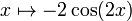$x \mapsto -2\cos(2x)$$n^{th}$ derivative$2^{n-1}$ times an expression that is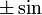$\pm \sin$ or$\pm \cos$ of$2x$, depending on the remainder of$n$ mod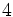$4$
antiderivative$x \mapsto \frac{x}{2} - \frac{\sin(2x)}{4} + C$
mean value over a period 1/2
expression as a sinusoidal function plus a constant function$(1/2) - \cos(2x)/2$
important symmetries even function
more generally, miror symmetry about any vertical line of the form$x = n\pi/2$,$n$ an integer.
Also, half turn symmetry about all points of the form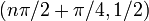$(n\pi/2 + \pi/4,1/2)$.
interval description based on increase/decrease and concave up/down For each integer$n$, the interval from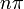$n\pi$ to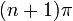$(n+1)\pi$ is subdivided into four pieces:$(n\pi, n\pi + \pi/4)$: increasing and concave up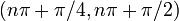$(n\pi + \pi/4,n\pi + \pi/2)$: increasing and concave down$(n\pi + \pi/2,n\pi + 3\pi/4)$: decreasing and concave down,$(n\pi + 3\pi/4,(n+1)\pi)$: decreasing and concave up
power series and Taylor series The power series about 0 (which is hence also the Taylor series) is$\sum_{k=1}^\infty \frac{(2)^{2k-1}(-1)^{k-1}x^{2k}}{k!}$
It is a globally convergent power series.

## Integration

### First antiderivative: using double angle formula

We use the identity:$\! \sin^2x = \frac{1 - \cos(2x)}{2}$

We can now do the integration:$\! \int \sin^2x \, dx = \int \frac{1 - \cos(2x)}{2} \, dx = \int \frac{1}{2} \, dx - \int \frac{\cos(2x)}{2} \, dx = \frac{x}{2} - \frac{\sin(2x)}{4} + C$

===First antiderivative: using integration by parts:

We rewrite$\sin^2x = (\sin x)(\sin x)$ and integrate by parts:$\int \sin^2x \, dx = (\sin x)(-\cos x) - \int (\cos x)(-\cos x) \, dx = -\sin x \cos x + \int \cos^2 x \, dx$

We now rewrite$\cos^2x = 1 - \sin^2x$ and obtain: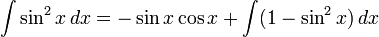$\int \sin^2x \, dx = -\sin x \cos x + \int (1 - \sin^2x) \, dx$

Setting$I$ to be a choice of antiderivative so that the above holds without any freely floating constants, we get: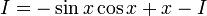$I = -\sin x \cos x + x - I$

Rearranging, we get: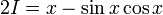$2I = x - \sin x \cos x$

This gives:$I = \frac{x - \sin x \cos x}{2}$

So the general antiderivative is: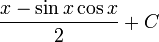$\frac{x - \sin x \cos x}{2} + C$

Using the double angle sine formula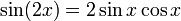$\sin(2x) = 2 \sin x \cos x$, we can verify that this matches with the preceding answer.

### Definite integrals

The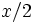$x/2$ part in the antiderivative signifies that the linear part of the antiderivative of$\sin^2$ has slope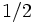$1/2$, and this is related to the fact that$\sin^2$ has a mean value of$1/2$ on any interval of length equal to the period. It is in fact clear that the function is a sinusoidal function about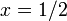$x = 1/2$.

Thus, we have:$\int_a^{a + n\pi} \sin^2x \, dx = \frac{n\pi}{2}$

where$n$ is an integer.

### Higher antiderivatives

It is possible to antidifferentiate$\sin^2$ more than once. The$n^{th}$ antiderivative is the sum of a polynomial of degree$n$ and a trigonometric function with a period of$\pi$.